# Feasibility and Accuracy Analysis of Input Power Estimation for Boost PFC Converters with Additional SensorsGet valuable resources straight to your inbox - sent out once per month

We value your privacy

## Introduction

PFC converters are widely used in the industry to provide DC output voltage from the main lines, and to maintain a high power factor (PF) and low current distortion at the same time. In some of these applications, such as telecom, server, workstation power supplies, and plug-in electric vehicles, the active input power needs to be measured in real time in order to monitor energy consumption, as well as to improve system efficiency and achieve intelligent system management. Dedicated voltage and current sensors are commonly adopted in front of the bridge rectifier to implement this power metering function. However, regardless of whether the additional sensors are shunt-based or Hall-effect based, they all add considerable cost, complexity, and power consumption to these power supplies.

According to the basic modeling of boost PFC converters, PFC controllers usually samples the input voltage, the output voltage, and the inductor current to control the switching frequency and the duty cycle. In this way, the output power is regulated and the input current is simultaneously shaped to achieved high PF and low total harmonic distortion (THD). Therefore, PFC controllers typically have the raw information that can be used for power estimation. Given that digital controllers have been widely adopted in the industry[2, 3, 4], this information is commonly accessible in real time through digital communication interfaces from PFC controllers. This creates a possible approach to monitor input power via indirect calculation instead of the conventional power metering implemented by additional voltage and current sensors.

Several key factors must be taken into consideration in order to get an estimation of the actual input power with acceptable accuracy. First, the passive components on the input, such as electro-magnetic compliance (EMC) components and the bridge rectifiers, make a difference between the power regulated in the boost converter and the actual input current. However, this is not the main problem, as the extra power introduced by passive components is straightforward to be modeled. In other words, it can be directly calculated based on the designed circuit parameters, especially considering that high-frequency distortions are not the primary concern for the fundamental power metering requirements. However, some non-ideal effects introduce more complicated influences on the accuracy of inductor current estimation. The turn-on and turn-off delays deviate the actual inductor current and the switching frequency from the control targets. The parasitic oscillation in DCM introduces another variable to the inductor current. In addition, these influences all vary with operating conditions. Most practical applications have adopted a multi-mode control scheme[6, 7, 8, 9], due to its superior light-load performance over a conventional critical mode (CrM) control scheme or fixed-frequency continuous conduction mode (CCM) control scheme. In order to accurately estimate power, those non-ideal effects must be considered under various operating conditions. Based on the observability of the PFC model, the PFC control scheme can be implemented even without sensing the inductor current[11, 12]. Therefore, the current error introduced by the delays can also be compensated based on proper modeling of the other system states and parameters, e.g. the input and output voltage, and the PFC inductor. On the other hand, the parasitic oscillation in DCM can be modeled in the time domain[13, 14], based on which the corresponding error in each switching can also be deduced.

In this paper, the influences of these effects are analyzed in detail based on a multi-mode PFC control scheme, and an improved, easy-to-implement algorithm for input power estimation is proposed to achieve accurate active power estimation across a wide operating range. A prototype with 400W of rated power has been built based on the HR1211GY, a digital PFC and LLC combo controller. The controller implements a multi-mode PFC control scheme that smoothly switches between CCM and DCM operation. With the experimental results based on such a mainstream control scheme in the industry, the feasibility and the universal applicability of the proposed estimation approach was verified.

## Multi-Mode PFC Control Scheme

A boost converter with typical multi-mode PFC control scheme, as shown in Fig.1, enables smooth transition between CCM and DCM to achieve high efficiency across a wide operating range. Operating in CCM can minimize both the peak current and RMS current under heavy-load conditions, which helps reduce the size of the magnetic components and makes it suitable for higher-power applications with good efficiency. Operating in DCM with a reduced switching frequency can minimize switching loss to achieve better power saving under light-load conditions. Furthermore, the hybrid operation of CCM and DCM under medium-load conditions optimizes the balance between conduction loss and switching loss to achieve better average efficiency. This hybrid is the emerging control scheme, and has been well recognized in the industry recently, because both full-load efficiency and light-load efficiency are equally important for most practical applications.

The control scheme, as shown in Fig.1, samples the output voltage VO and compares it to the output reference VO_REF to derive the internal compensation state vCOMP(n) through the PI loop regulator. Accordingly, an inner current loop generates the reference for the inductor current control, as in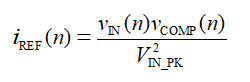Where vIN(n) is the sampled instant input voltage, and VIN_PK is the peak voltage in a line cycle.

By comparing 2iREF(n) to the inductor peak current iPK(n), which can be sampled right before the MOSFET turns off during each switching cycle, the control scheme is able to determine whether the converter should be operating in CCM or DCM. If it operates in CCM, the switching frequency fS is regulated at the maximum switching frequency fS_MAX as a steady state. And in order to keep the average current in every switching cycle equal to iREF(n), the MOSFET always turns on when the inductor current iL decreases to the targeted valley iVally, as in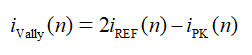At the same time, in order to guarantee the magnetizing balance of the PFC inductor, the on-time TON(n) is controlled as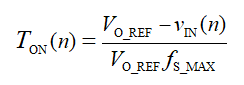If it operates in DCM, the on-time is still controlled in the same way, but the switching frequency is reduced to keep the average current equal to iREF(n). In general, the switching frequency is controlled as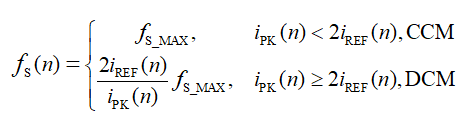In this way, the average iL in one switching cycle is always equal to iREF. Ideally, iREF can be used to calculate the input power. However, as shown in the following sections, without compensating for the non-ideal effects, estimation accuracy cannot be guaranteed.

## Current Control Error Caused by Parasitic Effects

The main parasitic effects that lead to error between the actual current and the control target in the boost converters are switching delays and current oscillation. The effects are related to converter parameters and vary with operating conditions.

In CCM, as shown in Fig.2, the error is mainly introduced via the turn-on delay TD_ON and the turn-off delay TD_OFF. The turn-on delay results in an undershoot below the targeted current valley, while the turn-off delay deviates the sampling point away from the actual peak point on the inductor current. The average error in one switching cycle can be calculated as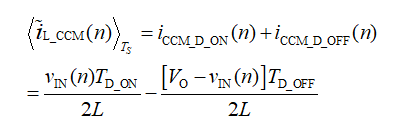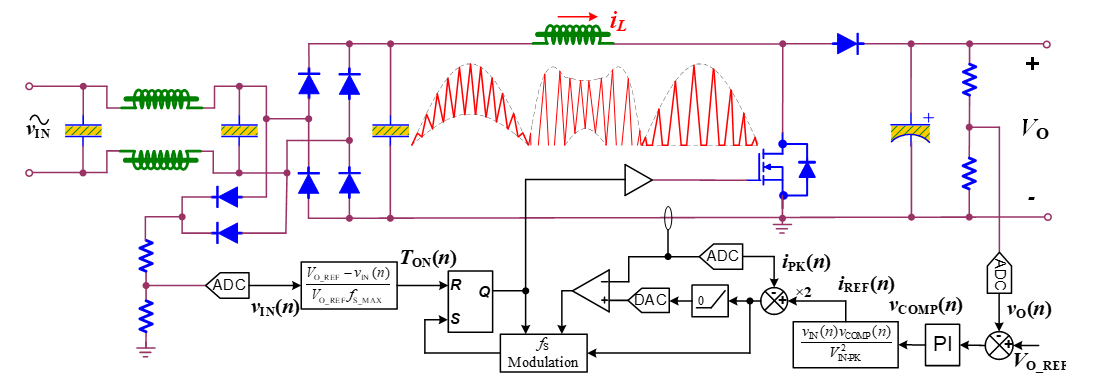Figure 1: Boost Converter with a Multi-Mode PFC Control Scheme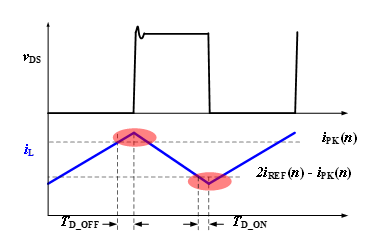Figure 2: Main Causes of Current Error in CCM

Where L is inductance of the PFC inductor.

In DCM, as shown in Fig.3, the inductor current always starts from zero, so the turn-on delay does not affect the inductor current anymore. However, the turn-off delay still has a similar effect on the peak sampling of the inductor current. The error model is different from that in CCM, because it has influence on both the peak and duty of the current. The average error in one switching cycle caused by the turn-off delay in DCM can be obtained asIn addition, DCM oscillation is another main cause of the current error. When the PFC inductor current drops to zero, the inductor oscillates with the equivalent parasitic capacitance of the MOSFET and the freewheeling diode. The initial states of the oscillation are with the zero inductor current and the drain-source voltage vDS at VO. Due to the clamping effect of the body diode of the MOSFET, there are two possible scenarios for the oscillation, depending on whether vDS oscillates to zero.

When vIN exceeds VO / 2, as shown in Fig.3 (a), the oscillation runs in a free damping way without being clamped on any point. Therefore, the average oscillating current in one switching cycle is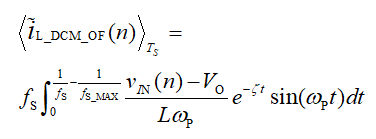Where ωP and ζ are the angular frequency and the damping coefficient of the oscillation.

When vIN is below VO / 2, as shown in Fig.3 (b), the oscillation is clamped by the body diode when vDS drops to zero. During the clamping period, the inductor current ramps up with a constant slew rate until the current polarity is reversed again. Then, free oscillation resumes. Therefore, the oscillation in this scenario is composed of three sections, and the durations of the first two can be respectively calculated as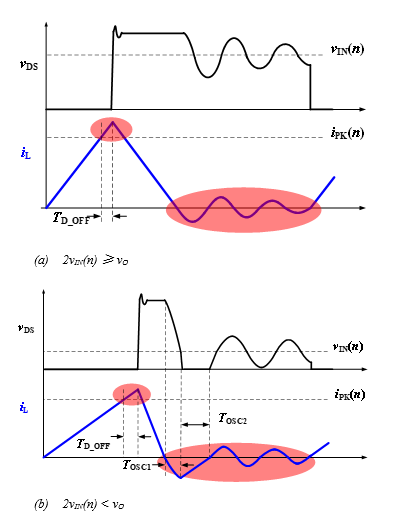Figure 3: Main Causes of Current Error in DCM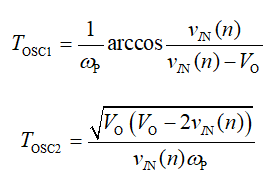Accordingly, the average oscillating current in one switching cycle can be obtained as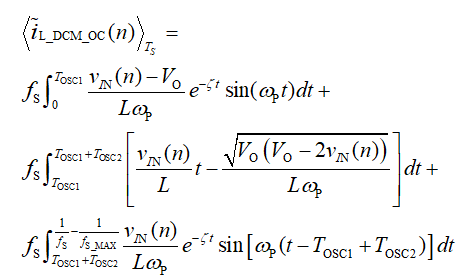Based on this, the general current error in DCM is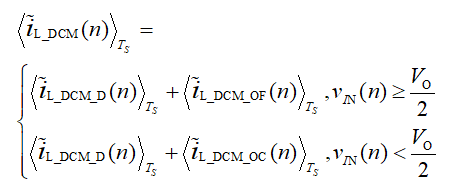The current error caused by the parasitic effects in each operating condition is formulated in (5) through (11). However, in order to make a universal current error compensation algorithm for practical application use, the boundaries between each condition must also be carried out. Assuming the transition angular between CCM and DCM in the first quarter of an AC input line cycle is θT, the input voltage and the reference current at the boundary conduction are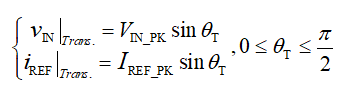Where IREF_PK is the peak reference current in a complete line cycle, and can be derived from vCOMP based on (1). The transition angulars in each quarter of an AC input line cycle are symmetrical to each other.

Since the inductor current is also in boundary conduction at the transition point, there is equation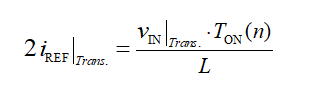Based on (2), (12), and (13), it can be deduced that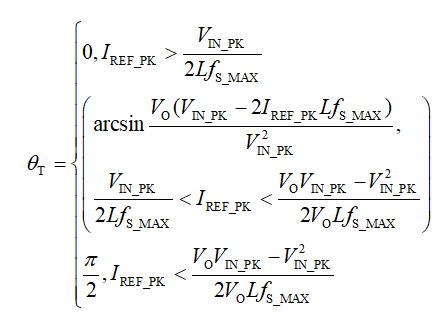Accordingly, the distribution of θT is plotted in Fig.4. When θT is equal to 0, the converter only operates in CCM. When θT is equal to π / 2, the converter only operates in DCM. When θT is between 0 and π / 2, the converter operates in both CCM and DCM during one AC input line cycle, and there is more portion of time in CCM as the load increases or the input voltage decreases.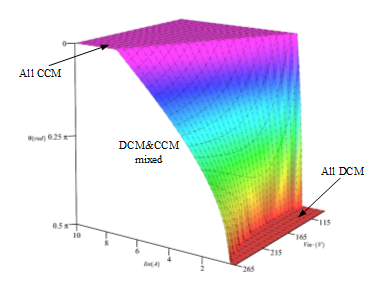Figure 4: CCM and DCM Distribution vs. Input and Load Conditions

## Active Input Power Estimation

The active input current can be accurately estimated by compensating the parasitic effects in various operating conditions, as shown by the analysis in Section III. In order to derive the active input power, the power loss introduced by the passive components on the input must also be addressed.

Taking the schematic illustrated in Fig.1 as a typical example, the passive components on the input of the PFC converter mainly include LC filters and bridge diodes. Considering that the reactive current flowing through the filter capacitors does not contribute to active power and that the leakage current of capacitors is small enough, the filter capacitors have little influence on the active power estimation. On the other hand, the voltage drop introduced by the bridge diodes and the parasitic resistance of the filter inductors leads to considerable power loss, and must be included in the power estimation. The input voltage in front of the passive components can be derived as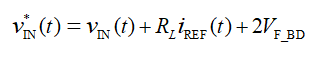Where VF_BD is the forward voltage of one bridge rectifier diode, RL is the total equivalent resistance of all the filter inductors, vIN(t) = VIN_PKsinωLt is the reconstructed voltage based on the sampled input voltage vIN(n) in the PFC control scheme, and ωL is the input line frequency.

Combing all the analysis above, the active input power can be estimated as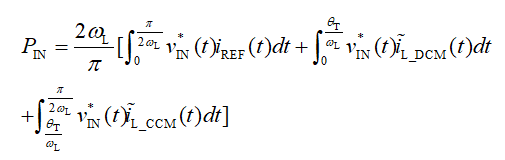## Experimental Verification

In order to verify the analysis and the proposed estimation of the input power, a 400W prototype has been built and tested. The prototype is designed and implemented based on the HR1211GY, a digital multi-mode PFC and LLC combo controller. The circuit and the control scheme of the PFC stage are the same as that shown in Fig.1, and Fig.5 shows a photo of the prototype. The specifications and key component parameters of the PFC stage include VIN_RMS = 90V - 265V, fL = 50Hz, RL = 100mΩ, VF_BD = 0.75V, VO = 400V, fS_MAX = 100kHz, L = 190µH, IPP60R099C7XKSA1 being the primary MOSFET, STPSC406D being the freewheeling diode, ωP = 5.93×106rad/s, TD_ON = 300ns, TD_OFF = 150ns. And the other instant states required for the power estimation, including vCOMP, VIN_PK and VO, are all accessible through the integrated UART interface of HR1211GY in real time.

The input current and the PFC inductor current waveforms are presented below. Fig.6 shows that the PFC converter fully operates in CCM under low-line and full-load conditions. The switching frequency is fixed as fS_MAX, and both the current peak and current valley are regulated in sinusoidal wave.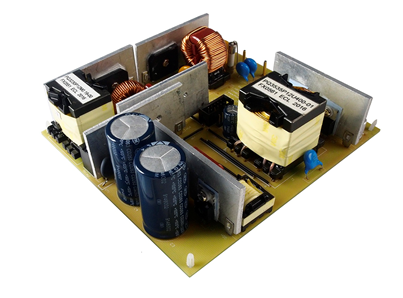Figure 5: 400W Prototype Based on the HR1211GY for the Experiment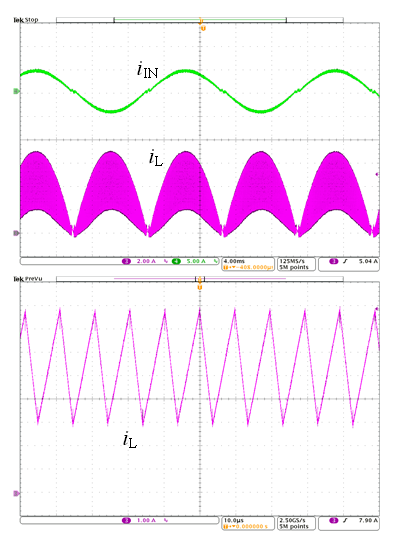Figure 6: Experimental Waveforms at VIN_rms = 110V and PO = 400W

Under high-line and full load conditions, the PFC converter operates in mixed CCM and DCM, as shown in Fig.7. The waveform shows the transition between CCM and DCM in the inductor current. In this way, the peak inductor current is lower at the peak of the input line, but the switching-cycle-average of the inductor current is still regulated to be sinusoidal by the controller.

Under light-load conditions, the PFC converter fully operates in DCM, as shown in Fig.8. The switching frequency becomes lower as the load decreases.

With the proposed power estimation approach, the input power from 10% to 100% load is calculated based on the parameters of this prototype illustrated above and the instant states read from the digital controller HR1211GY. Fig.9 shows the calculated results compared to the actual measured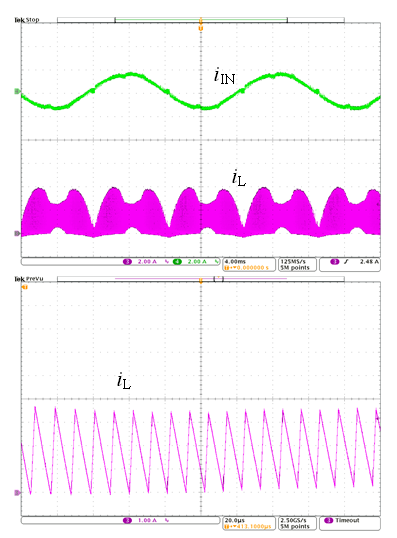Figure 7: Experimental Waveforms at VIN_rms = 230V and PO = 400W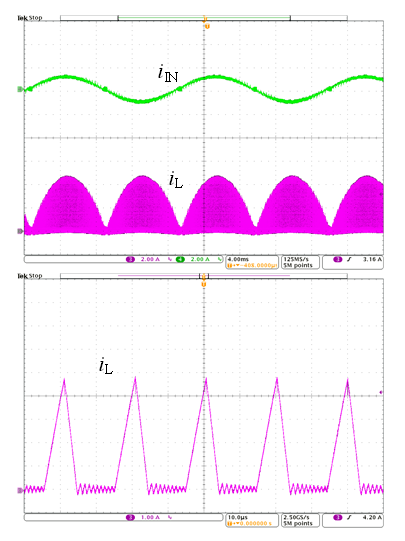Figure 8: Experimental waveforms at VIN_rms = 110V and PO = 100W

data using a WT310E power meter. It can be seen that the estimation error is smaller than 3% across a wide load range. In addition, different input conditions are covered by the same estimation algorithm.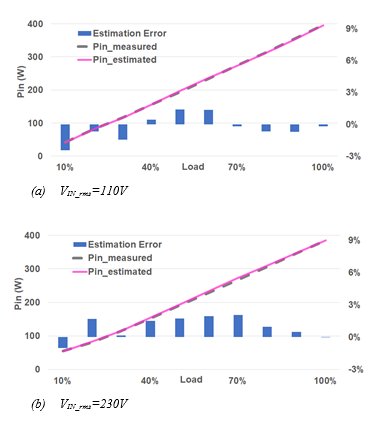Figure 9: Contrast Between the Calculated Input Power and the Measured Input Power

## Conclusion

This paper studied the feasibility of implementing active input power estimation without any additional sensors for boost PFC converter. The analysis in this paper accounts for the influence of parasitic effects, such as turn-on and turn-off delays, DCM oscillation, transition between DCM and CCM, and active power loss on passive components. Based on the mathematical modeling of the system and parasitic effects, an algorithm for accurately estimating the input power has been proposed. It takes the characteristics of the mainstream multi-mode PFC control scheme into consideration, and is able to support a wide operating range. The proposed estimation approach has been verified by experimental results on a 400W boost PFC prototype based on the HR1211GY. As digital PFC controllers are becoming more and more popular in practical applications, this study provides a possible approach to reduce system complexity and cost, as well as to improve reliability for power supply products in the future, by implementing basic power metering functions without adding any additional sensors.

## References

1. D. Simonetti, J. Vieira, and G. Sousa, “Modeling of the high-power factor discontinuous boost rectifiers,” in IEEE Trans. Ind. Electron., vol.46, no. 4, pp. 788–795, Aug. 1999.
2. K. De Gusseme, D. M. V. de Sype, A. P. Van den Bossche and J. A. Melkebeek, "Sample correction for digitally controlled boost PFC converters operating in both CCM and DCM," in Proc. IEEE Appl. Power Electron. Conf., 2003, pp. 389-395 vol.1.
3. [K. De Gusseme, D. M. V. de Sype, A. P. M. Van den Bossche and J. A. Melkebeek, "Digitally controlled boost power-factor-correction converters operating in both continuous and discontinuous conduction mode," in IEEE Trans. Ind. Electron., vol. 52, no. 1, pp. 88-97, Feb. 2005.
4. S. F. Lim and A. M. Khambadkone, "A Simple Digital DCM Control Scheme for Boost PFC Operating in Both CCM and DCM," in IEEE Trans. Ind. Appl. vol. 47, no. 4, pp. 1802-1812, July-Aug. 2011.
5. Y. Levron, H. Kim and R. W. Erickson, "Design of EMI Filters Having Low Harmonic Distortion in High-Power-Factor Converters," in IEEE Trans on Power Electron. vol. 29, no. 7, pp. 3403-3413, July 2014.
6. A. A. de Melo Bento and E. R. Cabral da Silva, "Hybrid One-Cycle Controller for Boost PFC Rectifier," in IEEE Trans. on Ind. Appl., vol. 45, no. 1, pp. 268-277, Jan.-Feb. 2009.
7. H. Kim, J. Kim, K. Park, H. Seong, G. Moon and M. Youn, "On/Off Control of Boost PFC Converters to Improve Light-Load Efficiency in Paralleled Power Supply Units for Servers," in IEEE Trans. Ind. Electron. vol. 61, no. 3, pp. 1235-1242, March 2014.
8. Y. Chen, Y. Chen and H. Chen, "On-time compensation method for CRM/DCM Boost PFC converter," in Proc. IEEE Appl. Power Electron. Conf., 2013, pp. 3096-3100.
9. Y. Chen and Y. Chen, "Line Current Distortion Compensation for DCM/CRM Boost PFC Converters," in IEEE Trans. Power Electron. vol. 31, no. 3, pp. 2026-2038, March 2016.
10. M. Pahlevani, S. Pan, S. Eren, A. Bakhshai and P. Jain, "An Adaptive Nonlinear Current Observer for Boost PFC AC/DC Converters," IEEE Tran on Ind. Electron., vol. 61, no. 12, pp. 6720-6729, Dec. 2014.
11. V. M. Lopez-Martin, F. J. Azcondo and A. de Castro, "Current error compensation for current-sensorless power factor corrector stage in continuous conduction mode," IEEE 13th Workshop, COMPEL, 2012, pp. 1-8.
12. V. M. Lopez, F. J. Azcondo, A. de Castro and R. Zane, "Universal Digital Controller for Boost CCM Power Factor Correction Stages Based on Current Rebuilding Concept," IEEE Trans. Power Electron., vol. 29, no. 7, pp. 3818-3829, Jul. 2014.
13. Q. Li, K. Yao, J. Song, H. Xu, and Y. Han, “A series diode method of suppressing parasitic oscillation for boost PFC converter operated in discontinuous conduction mode,” IEEE Trans. Power Electron., vol. 33, no. 1, pp. 407–424, Jan. 2018.
14. K. Yao, H, Xu, Q. Li, Y. Han, and K. Yun, “Detailed oscillation analysis and parameter selection principle for boost PFC converter with RC snubber operated in DCM,” IEEE Trans. Power Electron., vol. 34, no. 4, pp. 3348–3369, Apr. 2019.

### Did you find this interesting? Get valuable resources straight to your inbox - sent out once per month!

Get technical support